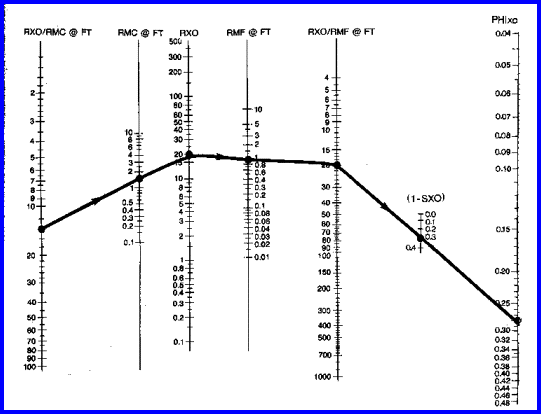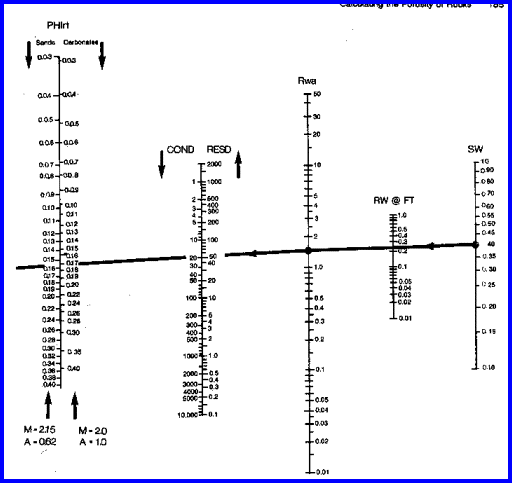Porosity from RESISTIVITY LOGSCAUTIONThe methods presented below provide a mechanism for analyzing ancient logs by computer. Experience has shown them to work well provided some control is exercised on the mud filtrate and water resistivity values upon which they depend. This is done by comparing results to cores or more modern log suites in the same formations nearby. When presented by computer, the results will not appear graphically to be any different or any less accurate than the most sophisticated multi-log analysis. Therefore, a warning note should be annotated on the results.

These porosity methods also rely on a knowledge  of SXO or SW, which cannot usually be derived accurately prior to knowing the correct porosity. Thus, if no other porosity method is available, these methods could give misleading results, with porosity being too low in hydrocarbon bearing zones.

References:
1.  Electrical Resistivity Log as an Aid in Determining Some Reservoir Characteristics
G.E. Archie, Journal of Petroleum Technology, 1941

2.
Resistivity of Brine Saturated Sands in Relation of Pore Geometry
W.O. Winsauer, H.M. Shearin, P.H. Masson, and M. Williams. AIME, 1952

3. The Microlog - A New Electrical Logging Method for Detailed Determinations of Permeable Beds

H.G. Dolll AIME, 1950

4. Microlaterolog
H.G. Doll,
JPT, 1953Porosity from Microlog
Many older wells do not have porosity indicatimg logs, but may have a microlog. Porosity can be derived, but it should be calibrated against core or more modern logs in offset wells. The response equation is based on Archie's formation factor and water saturation equations.

Calculate porosity from the microlog if there is positive separation.
1: IF RES2 > RES1
2: THEN PHIml = 0.614 ((RMF@FT * KML) ^ 0.61) / (R2 ^ 0.75)
3: OTHERWISE PHIml = 0

Where:
KML = correction factor for mud cake effect (fractional)
PHIml = porosity from microlog (fractional)
RES1 = shallow microlog (1 inch) reading (ohm-m)
RES2 = deep microlog (2in) reading (ohm-m)
RMF@FT = mud filtrate resistivity (ohm-m)COMMENTS:
No shale correction can be applied, so use caution. Since there is seldom any positive separation in really shaly sands, these will not usually cause any problem, except understate the potential of some shaly sands.

This method works well in good hole conditions, and with medium to high porosity. It should be used only if no other porosity indicating log is available, which is common in wells drilled before 1957. More complicated programs are available which simulate the microlog butterfly chart, but this simpler formula works nearly as well.

The chart and one such program are shown below.Chart for Microlog Porosity MethodFORTRAN Code for Microlog Porosity MethodRECOMMENDED PARAMETERS: Mud Weight KML lb/gal kg/m3 frac 8 1000 1.000 10 1200 0.847 11 1325 0.708 12 1440 0.584 13 1550 0.488 14 1680 0.412 16 1920 0.380 18 2160 0.350NUMERICAL EXAMPLE:
1. Assume microlog data:
RES1 = 3 ohm-m
RES2 = 4 ohm-m
RMF@FT = 1.0 ohm-m
mud weight = 1200 kg/m3
KML = 0.847
PHIml = 0.614 * ((1.0 * 0.847) ^ 0.61) / (4 ^ 0.75) = 0.20Porosity From Shallow Resistivity Logs
Porosity from proximity log, microlaterolog, microspherically focused log, spherically focused log, short normal, shallow induction, or shallow laterolog can be determined and is often used when no other porosity log is available. It can also be used to check microlog porosity if no other check is available. The response equation is based on Archie's formation factor and water saturation equations.

4: PHIxo = (A / ((RXO / RMF@FT) * (SXO ^ N))) ^ (1 / M)

Where:
A = tortuosity exponent
M = cementation exponent
N = saturation exponent
PHIxo = porosity derived from shallow resistivity device (fractional)
RMF@FT = mud filtrate resistivity at formation temperature (ohm-m)
RXO = resistivity from shallow resistivity device (ohm-m)
SXO = water saturation in invaded zone (fractional)COMMENTS:
No shale corrections are applied, so use caution. This method is a last resort, since an assumption about SXO must be made. SXO cannot be calculated for this method since it requires knowledge of porosity. Shale corrected versions of this equation can be created by inverting one of the shale corrected saturation equations.

A nomograph for solving these equations is provided below.Chart for Shallow Resistivity Porosity MethodRECOMMENDED PARAMETERS:
Normal values for A, M, N and SXO
for sandstone A = 0.62 M = 2.15 N = 2.00
for carbonates A = 1.00 M = 2.00 N = 2.00
for water zone SXO = 1.00
for hydrocarbon zone with high porosity SXO = 0.60
for hydrocarbon zone with medium porosity SXO = 0.70
for hydrocarbon zone with low porosity SXO = 0.80
for heavy oil and tar sands, SXO = SW = 0.10 to 0.30NUMERICAL EXAMPLE:
1. Assume shallow resistivity data:
RXO = 20 ohm-m
RMF@FT = 1.0 ohm-m
A = 0.62
M = 2.15
N = 2.00
SXO = 1.00
PHIxo = (0.62 / ((20.0 / 1.0) * (1.0 ^ 2.0))) ^ (1 / 2.15) = 0.20

2. If zone was hydrocarbon bearing, assume:
SXO = 0.70
PHIxo = (0.62 / ((20.0 / 1.0) * (0.7 ^ 2.0))) ^ (1 / 2.15) = 0.28Porosity from Deep or Medium Resistivity Log
This method can only be applied in water bearing zones, although correction for hydrocarbon content can be made if water saturation is reasonably well known from other sources, such as offset wells or capillary pressure data.The response equation is based on Archie's formation factor and water saturation equations.

5: PHIrt = (A / ((RESD / RW@FT) * (SW ^ N))) ^ (1 / M)

Where:
A = tortuosity exponent
M = cementation exponent
N = saturation exponent
PHIrt = porosity from deep resistivity (fractional)
RESD = deep resistivity log reading (ohm-m)
RW@FT = formation water resistivity (ohm-m)
SW = water saturation in un-invaded zone (fractional)COMMENTS:
No shale corrections are applied, so use caution. This method is not usually used in hydrocarbon zones and is an absolute last resort. The result is often used in a porosity playback log (with SW = 1.00) to look for possible hydrocarbon zones by observing the separation between PHIrt and the other porosity logs. Shale corrected methods may be created from the various shale corrected saturation equations.

A nomograph to solve these equations is provided below.Chart for Deep Resistivity Porosity MethodRECOMMENDED PARAMETERS:
Normal values for A, M, N and SW:
for sandstone A = 0.62 M = 2.15 N = 2.00
for carbonates A = 1.00 M = 2.00 N = 2.00
for water zones SW = 1.00
for hydrocarbon zone with high porosity SW = 0.20
for hydrocarbon zone with medium porosity SW = 0.40
for hydrocarbons zone with low porosity SW = 0.60NUMERICAL EXAMPLE:
1. Assume deep resistivity data:
RESD = 5.0 ohm-m
RW@FT = 0.25 ohm-m
A = 0.62
M = 2.15
N = 2.00
SW = 1.00
PHIrt = (0.62 / ((5.0 / 0.25) * (1.0 ^ 2.0)) ^ (1 / 2.15) = 0.20

If SW = 0.40
PHIrt = (0.62 / ((5.0 / 0.25) * (0.4 ^ 2.0))) ^ (1 / 2.15) = 0.46

2. This last result suggests the zone could not be hydrocarbon bearing, otherwise the RESD value was incorrectly picked. Assume RESD = 50, then;
PHIrt = (0.62 / ((50 / 0.25) * (0.4 ^ 2.0))) ^ (1 / 2.15) = 0.16

This is a more reasonable result.ESTIMATING SXO and SW
The methods presented in this Chapter provide a mechanism for analyzing ancient logs by computer. Experience has shown them to work well provided some control is exercised on the mud filtrate and water resistivity values upon which they depend. This is done by comparing results to cores or more modern log suites in the same formations nearby. When presented by computer, the results will not appear graphically to be any different or any less accurate than the most sophisticated multi-log analysis. Therefore, a warning note should be annotated on the results.

These porosity methods also rely on a knowledge of SXO or SW, which cannot usually be derived accurately prior to knowing the correct porosity. Thus, if no other porosity method is available, these methods could give misleading results, with porosity being too low in hydrocarbon bearing zones.

If approximate porosity is known, water saturation (SW) can be estimated from the Buckle's PHIxSW method or the resistivity ratio method:
6: SW = KBUCKL / PHIestimatedPARAMETERS:

Sandstones                  Carbonates              KBUCKL

Very fine grain            Chalky                          0.120

Fine grain                   Cryptocrystalline           0.060

Medium grain              Intercrystalline             0.040

Coarse grain               Sucrosic                        0.020

Conglomerate             Fine vuggy                    0.010

Unconsolidated           Coarse vuggy                0.005

Fractured                    Fractured                      0.001

Use these parameters only if no other source exists.

Invaded zone saturation (SXO) can be estimated from:
7: SXO = (SW) ^ (1 / 5)

Where:
PHIestimated = estimated effective porosity (fractional)
KBUCKL = porosity saturation product (fractional)
SW = water saturation (fractional)
SXO = invaded zone water saturation (fractional)

This approach could be more accurate than the guidelines provided earlier for estimating SW and SXO. Shale corrections are not included, so care must be exercised in shaly sands.

Page Views ---- Since 01 Jan 2015
Copyright 2023 by Accessible Petrophysics Ltd.
CPH Logo, "CPH", "CPH Gold Member", "CPH Platinum Member", "Crain's Rules", "Meta/Log", "Computer-Ready-Math", "Petro/Fusion Scripts" are Trademarks of the Author